# Geometry: Translations on a Grid

In this worksheet, students translate points and shapes using the given right/left or up/down instruction.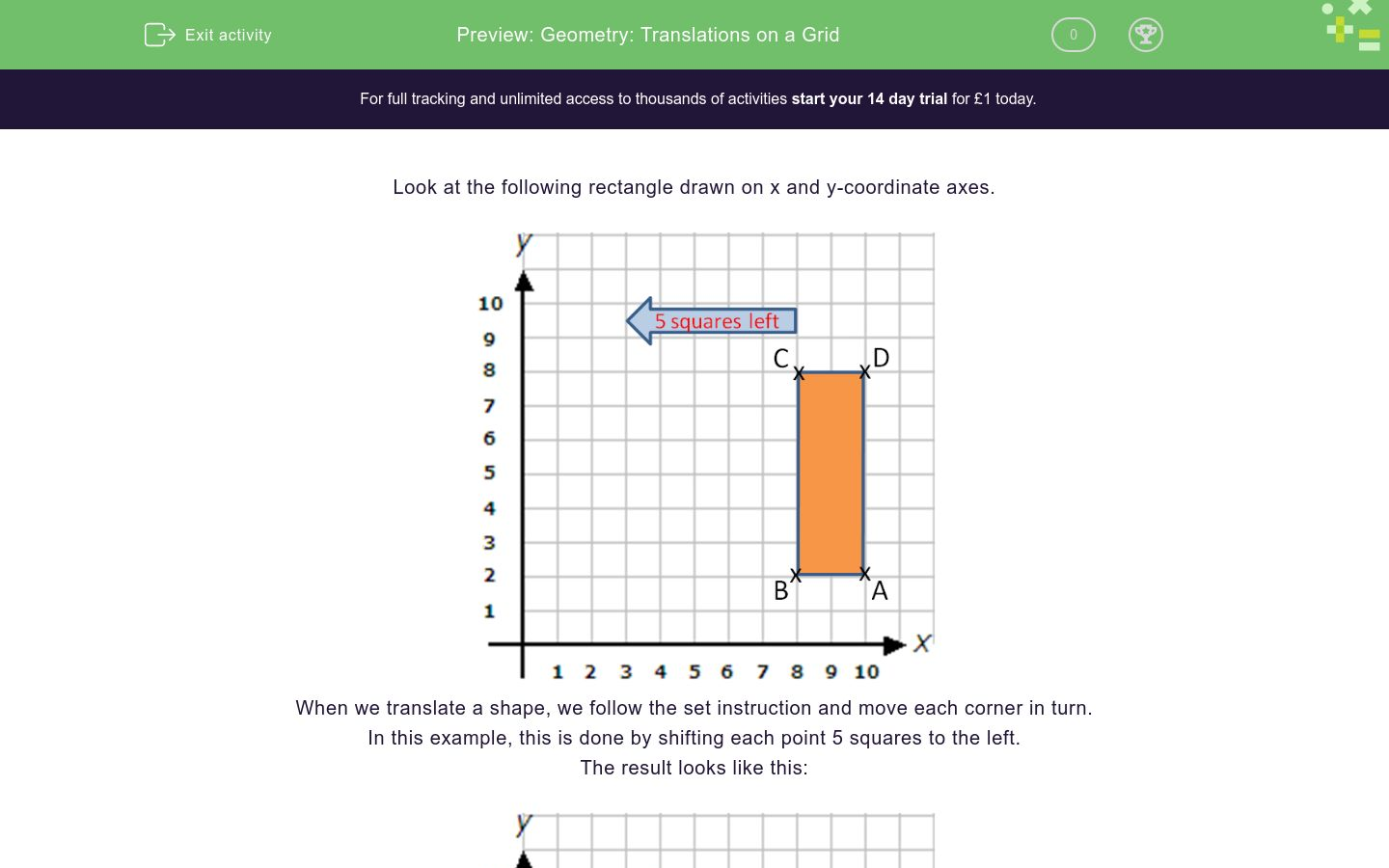Key stage:  KS 2

Curriculum topic:   Geometry: Position and Direction

Curriculum subtopic:   Draw/Translate Shapes

Difficulty level:### QUESTION 1 of 10

Look at the following rectangle drawn on x and y-coordinate axes.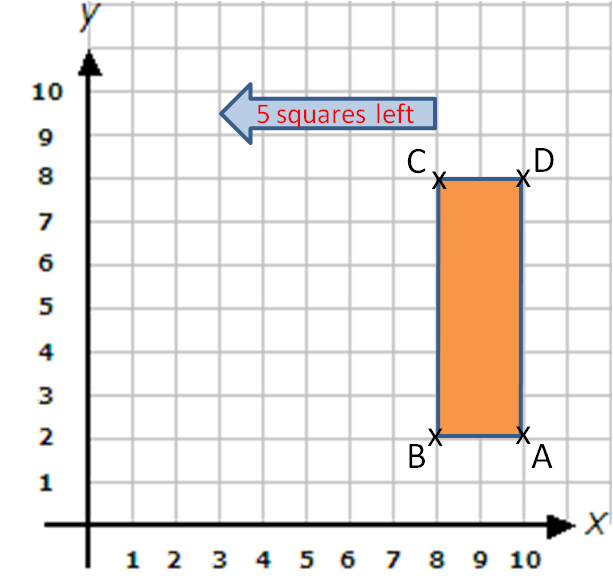When we translate a shape, we follow the set instruction and move each corner in turn.

In this example, this is done by shifting each point 5 squares to the left.

The result looks like this:Rectangle ABCD has been translated 5 squares to the left to form Rectangle A'B'C'D'.

Look at this diagram, which shows a rectangle ABCD, which is to be translated 6 squares to the left to give rectangle A'B'C'D'.

The point A has been translated to the point A'.

Write down the coordinates of B'. (Remember to use brackets when writing coordinates).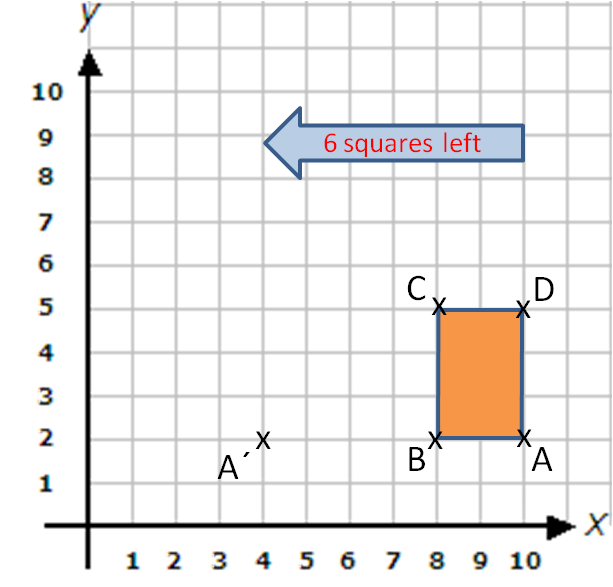Look at this diagram, which shows a rectangle ABCD, which is to be translated 6 squares to the left to give rectangle A'B'C'D'.

The point A has been translated to the point A'.

Write down the coordinates of C'. (Remember to use brackets when writing coordinates).Look at this diagram, which shows a rectangle ABCD, which is to be translated 6 squares to the left to give rectangle A'B'C'D'.

The point A has been translated to the point A'.

Write down the coordinates of D'. (Remember to use brackets when writing coordinates).Look at this diagram, which shows a rectangle ABCD, which is to be translated 5 squares up to give rectangle A'B'C'D'.

Write down the coordinates of A'.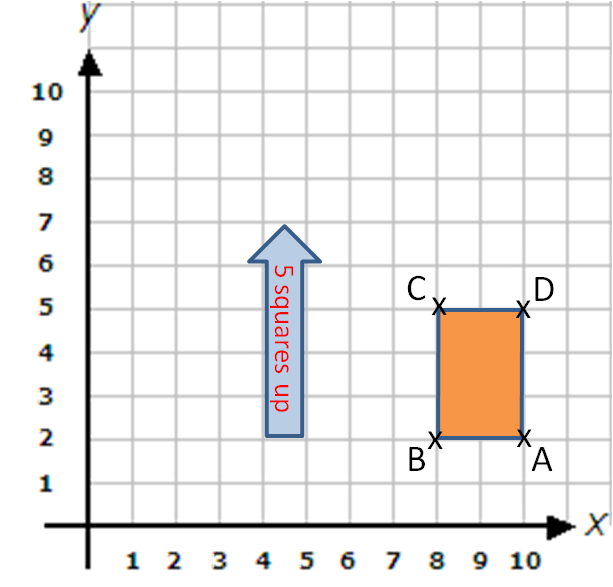Look at this diagram, which shows a rectangle ABCD, which is to be translated 5 squares up to give rectangle A'B'C'D'.

Write down the coordinates of B'.Look at this diagram, which shows a rectangle ABCD, which is to be translated 5 squares up to give rectangle A'B'C'D'.

Write down the coordinates of C'.Look at this diagram, which shows a rectangle ABCD, which is to be translated 5 squares up to give rectangle A'B'C'D'.

Write down the coordinates of D'.Look at this diagram, which shows a rectangle ABCD, which is to be translated 5 squares up and 6 squares to the left to give rectangle A'B'C'D'.

The point A has been translated to the point A'.  Notice that we count 5 squares up from A and then 6 squares to the left to get A'.

Write down the coordinates of B'.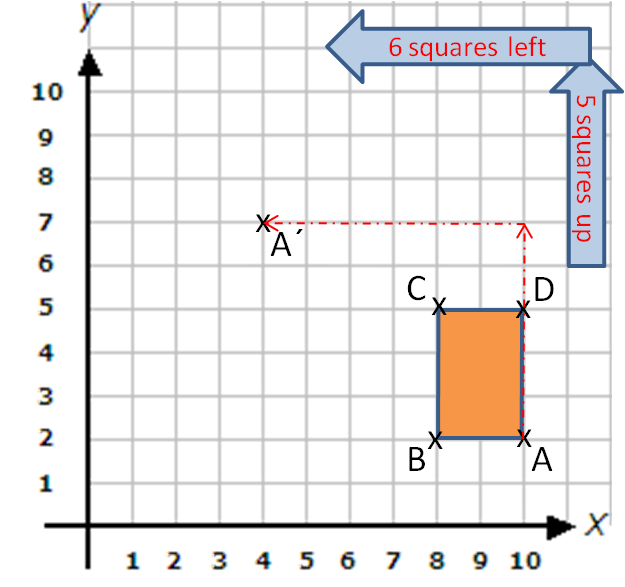Look at this diagram, which shows a rectangle ABCD, which is to be translated 5 squares up and 6 squares to the left to give rectangle A'B'C'D'.

The point A has been translated to the point A'.  Notice that we count 5 squares up from A and then 6 squares to the left to get A'.

Write down the coordinates of C'.Look at this diagram, which shows a rectangle ABCD, which is to be translated 5 squares up and 6 squares to the left to give rectangle A'B'C'D'.

The point A has been translated to the point A'.  Notice that we count 5 squares up from A and then 6 squares to the left to get A'.

Write down the coordinates of D'.• Question 1

Look at this diagram, which shows a rectangle ABCD, which is to be translated 6 squares to the left to give rectangle A'B'C'D'.

The point A has been translated to the point A'.

Write down the coordinates of B'. (Remember to use brackets when writing coordinates).(2,2)
(2, 2)
EDDIE SAYS
Move B six squares to the left to get (2, 2).
• Question 2

Look at this diagram, which shows a rectangle ABCD, which is to be translated 6 squares to the left to give rectangle A'B'C'D'.

The point A has been translated to the point A'.

Write down the coordinates of C'. (Remember to use brackets when writing coordinates).(2,5)
(2, 5)
EDDIE SAYS
Move C six squares to the left to get (2, 5).
• Question 3

Look at this diagram, which shows a rectangle ABCD, which is to be translated 6 squares to the left to give rectangle A'B'C'D'.

The point A has been translated to the point A'.

Write down the coordinates of D'. (Remember to use brackets when writing coordinates).(4,5)
(4, 5)
EDDIE SAYS
Move D six squares to the left to (4, 5).
• Question 4

Look at this diagram, which shows a rectangle ABCD, which is to be translated 5 squares up to give rectangle A'B'C'D'.

Write down the coordinates of A'.(10,7)
(10, 7)
EDDIE SAYS
Move A five squares up to (10, 7).
• Question 5

Look at this diagram, which shows a rectangle ABCD, which is to be translated 5 squares up to give rectangle A'B'C'D'.

Write down the coordinates of B'.(8,7)
(8, 7)
EDDIE SAYS
Move B five squares up to (8, 7).
• Question 6

Look at this diagram, which shows a rectangle ABCD, which is to be translated 5 squares up to give rectangle A'B'C'D'.

Write down the coordinates of C'.(8,10)
(8, 10)
EDDIE SAYS
Move C five squares up to (8, 10).
• Question 7

Look at this diagram, which shows a rectangle ABCD, which is to be translated 5 squares up to give rectangle A'B'C'D'.

Write down the coordinates of D'.(10,10)
(10, 10)
EDDIE SAYS
Move D up five squares to (10, 10).
• Question 8

Look at this diagram, which shows a rectangle ABCD, which is to be translated 5 squares up and 6 squares to the left to give rectangle A'B'C'D'.

The point A has been translated to the point A'.  Notice that we count 5 squares up from A and then 6 squares to the left to get A'.

Write down the coordinates of B'.(2,7)
(2, 7)
EDDIE SAYS
Move B five squares up and six squares to the left to get (2, 7).
• Question 9

Look at this diagram, which shows a rectangle ABCD, which is to be translated 5 squares up and 6 squares to the left to give rectangle A'B'C'D'.

The point A has been translated to the point A'.  Notice that we count 5 squares up from A and then 6 squares to the left to get A'.

Write down the coordinates of C'.(2,10)
(2, 10)
EDDIE SAYS
Move C five squares up and six squares to the left to get (2, 10).
• Question 10

Look at this diagram, which shows a rectangle ABCD, which is to be translated 5 squares up and 6 squares to the left to give rectangle A'B'C'D'.

The point A has been translated to the point A'.  Notice that we count 5 squares up from A and then 6 squares to the left to get A'.

Write down the coordinates of D'.(4,10)
(4, 10)
EDDIE SAYS
Move D five squares up and six squares to the left to get (4, 10).
---- OR ----

Sign up for a £1 trial so you can track and measure your child's progress on this activity.

### What is EdPlace?

We're your National Curriculum aligned online education content provider helping each child succeed in English, maths and science from year 1 to GCSE. With an EdPlace account you’ll be able to track and measure progress, helping each child achieve their best. We build confidence and attainment by personalising each child’s learning at a level that suits them.

Get started